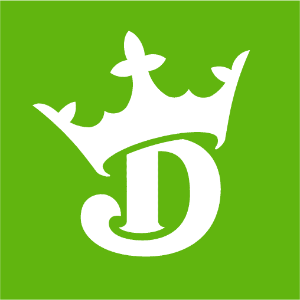DraftKings 2019 Super Bowl Odds
+250
2.5 to 1Ravens
12.7% implied probability

+400
4 to 1Patriots
8.9% implied probability

+500
5 to 1Saints
7.4% implied probability

+750
7.5 to 1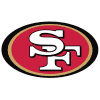49ers
5.2% implied probability

+800
8 to 1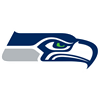Seahawks
4.9% implied probability

+1100
11 to 1Chiefs
3.7% implied probability

+1600
16 to 1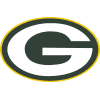Packers
2.6% implied probability

+2500
25 to 1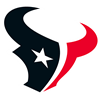Texans
1.7% implied probability

+2500
25 to 1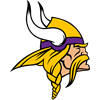Vikings
1.7% implied probability

+3000
30 to 1Cowboys
1.4% implied probability

+4000
40 to 1Bills
1.1% implied probability

+5000
50 to 1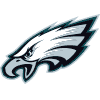Eagles
0.9% implied probability

+6600
66 to 1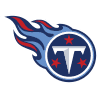Titans
0.7% implied probability

+8000
80 to 1Steelers
0.5% implied probability

+10000
100 to 1Rams
0.4% implied probability

+12500
125 to 1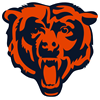Bears
0.4% implied probability

+15000
150 to 1Raiders
0.3% implied probability

+15000
150 to 1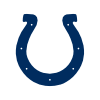Colts
0.3% implied probability

+35000
350 to 1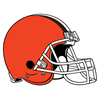Browns
0.1% implied probability

+50000
500 to 1Panthers
0.1% implied probability

+50000
500 to 1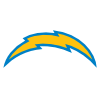Chargers
0.1% implied probability

+50000
500 to 1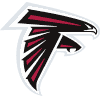Falcons
0.1% implied probability

+50000
500 to 1Buccaneers
0.1% implied probability

+75000
750 to 1Broncos
0.1% implied probability

+75000
750 to 1Cardinals
0.1% implied probability

+75000
750 to 1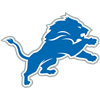Lions
0.1% implied probability

+100000
1000 to 1Redskins
0.0% implied probability

+100000
1000 to 1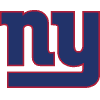Giants
0.0% implied probability

+100000
1000 to 1Jets
0.0% implied probability

+100000
1000 to 1Jaguars
0.0% implied probability

+500000
5000 to 1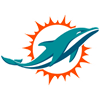Dolphins
0.0% implied probability